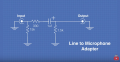# Calculating voltage attenuation in passive resistor circuit

#### eljeffe

Joined Sep 28, 2017
39
Howdy
Recently I was trying to record a line-level signal into my phone input jack, and of course the level was too loud. I found a very good video (YouTube link) that provides a simple passive resistor circuit to drop the level.
How can I calculate the voltage drop that is happening via that 330ohm resistor?

Here is the circuit:This almost looks like the normal voltage divider circuit but I'm not entirely sure. Would the 330ohm resister be R1 and the 1.5kohm resistor be R2, such as in this calculator? http://www.ohmslawcalculator.com/voltage-divider-calculator

How important are the values for the resistors to ground? What happens if I start modifying those?

Thanks for any insight

#### Papabravo

Joined Feb 24, 2006
13,726
Howdy
Recently I was trying to record a line-level signal into my phone input jack, and of course the level was too loud. I found a very good video (YouTube link) that provides a simple passive resistor circuit to drop the level.
How can I calculate the voltage drop that is happening via that 330ohm resistor?

Here is the circuit:
View attachment 208364

This almost looks like the normal voltage divider circuit but I'm not entirely sure. Would the 330ohm resister be R1 and the 1.5kohm resistor be R2, such as in this calculator? http://www.ohmslawcalculator.com/voltage-divider-calculator

How important are the values for the resistors to ground? What happens if I start modifying those?

Thanks for any insight
The easiest way to see what is going on is with a simulator like LTspice which you can download for free. It has a learning curve so maybe keep that in mind for the future. Since the first 15K resistor is much larger than the others in the circuit we are going to ignore it for now. The next thing you should do is compute the impedance of the 1 uF capacitor at audio frequencies. Do you know how to do that? Then we can deal with the 330 and the 1.5K. It is basically a high pass filter from 10 Hz to above the audio range. The passband attenuation is determined by the 330 and the 1.5 K and is about 0.81 or -1.745 dB. The solid green line is the magnitude response and the dotted green line is the phase response.

Last edited:

#### eljeffe

Joined Sep 28, 2017
39
The next thing you should do is compute the impedance of the 1 uF capacitor at audio frequencies. Do you know how to do that?
I do not. Care to enlighten me?
I have spice and have used it before via eagle, so I could try that for sure. I was just trying to see if my basic understanding was there. Using the calculator I linked to, I got the same result (~0.82%) as you so that is encouraging.

#### Papabravo

Joined Feb 24, 2006
13,726
The impedance of the capacitor is frequency dependent. In this circuit, its purpose is to block DC and low frequency AC. For this circuit, low frequency is from DC to a couple of hundred Hz. the impedance of the capacitor is given by:

$X_C\;=\;\frac{1}{2\pi fC}$

The following table has a my calculations for some typical values
 f​ Xc​ 10​ 15915.5​ 100​ 1591.5​ 200​ 795.8​ 300​ 530.5​ 400​ 397.9​ 3000​ 53.1​